# High School Chemistry : Elements and Atoms

## Example Questions

← Previous 1 3 4 5

### Example Question #1 : Identifying Elements

Which of the following defines the identity of an element?

Number of electron shells

Number of nucleons

Number of protons

Number of electrons

Number of neutrons

Number of protons

Explanation:

The identity of an element is determined by the number of protons. One cannot alter the number of protons without altering the identity of the element. By adding a proton, the atomic number increases by one and the element identity changes.

Number of neutrons can be altered to create isotopes. Number of electrons can be altered to create ions.

### Example Question #2 : Elements And Compounds

What element could contain seven protons, eight neutrons, and seven electrons?

Nitrogen

Depending on isotope and ionization, any of these could have the given configuration

Oxygen

Fluorine

Carbon

Nitrogen

Explanation:

An element is defined by its number of protons. Varying the number of neutrons will change the isotope of an element and varying the number of electrons will create an ion, but changing the number of protons changes the identity of the element itself. Nitrogen has atomic number seven, and is the only element that can have seven protons. The given configuration is for an isotope of nitrogen. Since there are eight neutrons, this atom would be for nitrogen-15.

### Example Question #3 : Elements And Compounds

Which of the following molecules consists of only one element?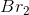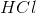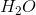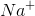Explanation:

Elements are shown on the periodic table. Compounds are made when one or more element is combined to form a molecule. The smallest unit of an element is an atom, while the smallest unit of a compound is a molecule.

Of the given answer choices, onlysatisfies the question criteria. This is the formula for a molecule of bromine gas, made of two bromine atoms.is a sodium ion, representing one atom of sodium that has lost an electron to gain a positive charge. Though it contains only one element, it is not a molecule.is a single carbon atom, and also does not represent a molecule.is the formula for hydrochloric acid andis the formula for water. Both of these compounds are composed of molecules, but hydrochloric acids contains both hydrogen and chlorine atoms, and water contains both hydrogen and oxygen.

### Example Question #1 : Identifying Elements

Is it possible to form a compound from only one element?

Yes; benzene rings contain only carbon

No; a compound must contain multiple elements

Yes; compounds and elements are synonyms

Yes; diatomic gases are compounds that consist of only one element

Yes; water is a compound that consists of only one element

Yes; diatomic gases are compounds that consist of only one element

Explanation:

Elements are defined by the number of protons in a given atom, and the atom is the smallest unit of an element. In contrast, a compound is defined by the identity and organization of multiple atoms, with the smallest unit of a compound being a molecule.

Some compounds do contain only one element, such as diatomic gases or graphite. Benzene rings are composed of carbon and hydrogen, while water molecules contain hydrogen and oxygen.

### Example Question #1 : Elements And Atoms

Which of the following atomic properties, if known, will reveal the identity of the element?

The atomic mass

The number of protons

More than one of these answers could allow us to identify the given atom

The number of neutrons

The number of electrons

The number of protons

Explanation:

There are two properties that can be used to identify an element: the atomic number or the number of protons in an atom.

The number of neutrons and number of electrons are frequently equal to the number of protons, but can vary depending on the atom in question. Different ions and isotopes will alter the number of neutrons and electrons, making these properties unreliable identifiers. Similarly, atomic mass will vary with the number of neutrons present and cannot be used to establish atomic identity.

### Example Question #1 : Identifying Elements

Which of the following phase labels are used for all diatomic elements?

(g)

(aq)

(l)

(r)

(s)

(g)

Explanation:

Diatomic elements are commonly referred to as diatomic gases because whenever they are by themselves, they bond to themselves (that is where the "2" subscript comes from). Anytime diatomic elements have that "2" subscript, they have the gas (g) phase label. For example,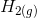.

### Example Question #1 : Elements And Atoms

What is the chemical symbol for potassium?

Pt

Po

K

P

Na

K

Explanation:

The symbol for potassium comes from the latin word for potassium, kalium. That is why the chemical symbol for potassium is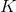.

### Example Question #1 : Identifying Elements

Which element also goes by its latin name, ferrum?

Iron

Platinum

Copper

Gold

Iron

Explanation:

The word for iron in latin is ferrum, thus, the chemical symbol for iron is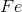because of its latin name.

### Example Question #1 : Elements And Atoms

Which element has 42 protons?

Mercury

Niobium

Molybdenum

Technetium

Gold

Molybdenum

Explanation:

Remember, the number of protons is equal to the atomic number of the element. Look at the periodic table and find the element with atomic number 42, it corresponds to molybdenum.

### Example Question #1 : Identifying Elements

Which element has 17 electrons?

Phosphorus

Sulfur

Chlorine

Argon

Silicon